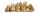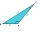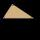# Expression of a variable from formula - problems - page 28

1. HectolitersHow deep is the pool if there are 2025 hectoliters of water and the bottom dimensions are a = 15 meters b = 7,5 meters and the water level is up to 9/10 (nine-tenths) of height.
2. Concentric circlesThere is given a circle K with a radius r = 8 cm. How large must a radius have a smaller concentric circle that divides the circle K into two parts with the same area?
3. Perimeter of a rectangleIf the perimeter of a rectangle is 114 meters and the length is twice the width plus 6 meters, what are the length and width?
4. GP membersThe geometric sequence has 10 members. The last two members are 2 and -1. Which member is -1/16?
5. ExpressionSolve for a specified variable: P=a+4b+3c, for a
6. TwentyTwenty rabbits are put in 4 cells so that there are different number of rabbits in each cell contains at least 3 rabbits. What is the largest possible number of rabbits in one cell
7. Area to perimeterCalculate circle circumference if its area is 254.34cm2
8. The volume 2The volume of a cube is 27 cubic meters. Find the height of the cube.
9. Rectangular trapezoidIn a rectangular trapezoid ABCD with right angles at vertices A and D with sides a = 12cm, b = 13cm, c = 7cm. Find the angles beta and gamma and height v.
10. AlgebraX+y=5, find xy (find the product of x and y if x+y = 5)
11. Ratio of volumesIf the heights of two cylindrical drums are in the ratio 7:8 and their base radii are in the ratio 4:3. What is the ratio of their volumes?
12. Calculate 3Calculate the cube volume whose edge is 3x-1,3x-1,3x-1
13. A rectangleA rectangle has an area of 36 cm2. What could the length and width of rectangle be?
14. Calculate 2Calculate the largest angle of the triangle whose side are 5.2cm, 3.6cm, and 2.1cm
15. Find the 2Find the term independent of x in the expansion of (4x3+1/2x)8
16. Expression with powersIf x-1/x=5, find the value of x4+1/x4
17. Rectangular fieldA rectangular field has a diagonal of length 169m. If the length and width are in the ratio 12:5. Find the dimensions of the field, the perimeter of the field and the area of the field.
18. Pile of sandA large pile of sand has been dumped into a conical pile in a warehouse. The slant height of the pile is 20 feet. The diameter of the base of the sand pile is 31 feet. Find the volume of the pile of sand.
19. ThunderstormThe height of the pole before the storm is 10 m. After a storm when they come to check it they see that on the ground from the pole blows part of the column. Distance from the pole is 3 meters. At how high was the pole broken? (In fact, a rectangular tria
20. BrakesThe braking efficiency of a passenger car is required to stop at 12.5 m at an initial speed of 40 km/h. What is the acceleration braking by brakes?

Do you have an interesting mathematical problem that you can't solve it? Enter it, and we can try to solve it.

To this e-mail address, we will reply solution; solved examples are also published here. Please enter e-mail correctly and check whether you don't have a full mailbox.